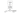## The Hypothesis Function

Our hypothesis function has the general form:

$\hat{y} = h_\theta(x) = \theta_0 + \theta_1 x$

The hypothesis function $h_\theta(x)$ is sometimes simply denoted as $h(x)$.

Note that this is like the equation of a straight line. We give to $h_\theta(x)$ values for $\theta_0$ and $\theta_1$ to get our estimated output $\hat{y}$.

We try to find proper values for $\theta_0$ and $\theta_1$ which provide the best possible "fit" or the most representative "straight line" through the data points mapped on the x-y plane.

A list of m training examples — $(x^{(i)},y^{(i)}); i=1,...,m$ — is called a training set. Our goal is, given a training set, to learn a hypothesis function h : X → Y so that h(x) is a “good” predictor for the corresponding value of y.

We will also use X to denote the space of input values, and Y to denote the space of output values. In this example, $X = Y = \mathbb{R}$.